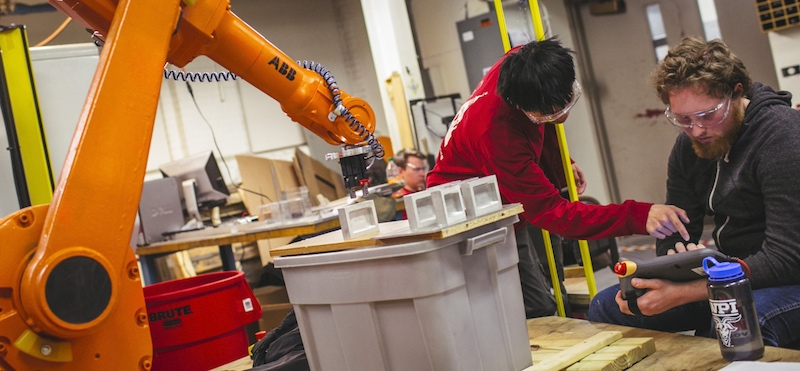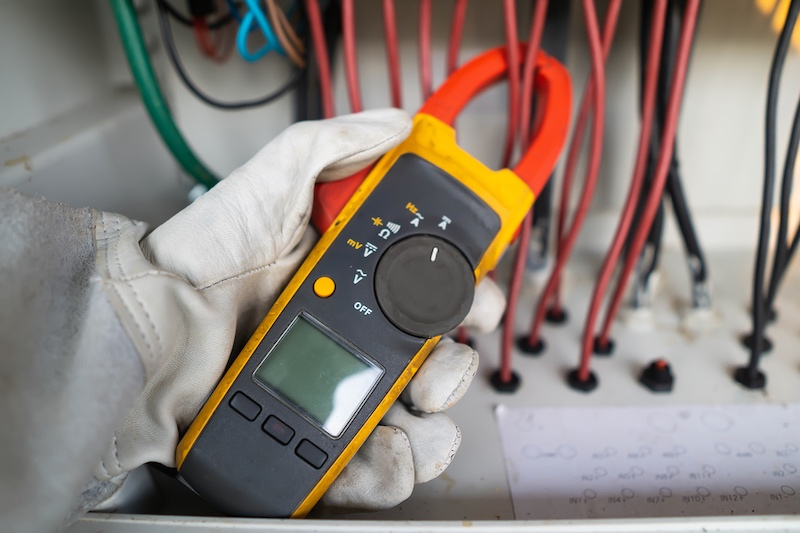Technical Article

# The Importance of Mathematics for Troubleshooting and Control Operations

April 20, 2021 by David Peterson

## From plant management to tuning your control system, there are a few specific math concepts you may need to know.

The field of mathematics is broad, with applications in nearly every imaginable field from medicine, business, technology, and data analysis. Whether you’re a control system designer, machine operator, maintenance technician, or automation engineer, there are some specific math concepts that you should know.

### Math Concepts for Mechatronics

In a discussion of mathematics with any technical audience, there is always apprehension to the idea that a calculator is not always necessary.##### Two industrial engineers working together to solve mathematical problems in their facility.

But in fact, most troubleshooting and analysis situations can be more efficiently solved with a rough calculation, rather than taking the time to solve an equation down to several decimal places of precision. The problem with this idea is that very few people memorize all the equations necessary to apply mental math for every situation.

Instead, focus on those situations that you encounter daily and become most familiar with quickly obtaining those reasonable solutions.

For machine operators and maintenance technicians, algebra is the most common field of mathematics required to find solutions. As always in math, there’s a lot to consider, including the situation, formula, and finding a solution. The following list details many of the common situations that can be aided by a mental understanding of how the math applies to daily control operations.

### Addition: Why Extreme Precision is Important

Addition is perhaps the most simple math application, but it can become immediately confusing when electrical terms are introduced. There are numerous situations in which addition is helpful.

Adding voltage drops in a series, such as the voltage of a wire, and a load, and a control device, can help determine the exact voltage input needs of a circuit. The same is said with resistances in series. When multiple circuits are being supplied from a single source, the current of all branch circuits must be added to determine the total current load.

Complexity is introduced when numbers of different sizes are used in one addition problem. In the previous voltage drop example, a 24-volt load may be added to a 3 millivolt drop in the series wire and a 1 millivolt drop in the switch. In such cases, the mental math could simply say ‘the total is just slightly more than 24 volts’.##### Two students studying industrial engineering working together to calculate and add movements to control this ABB robot. Image used courtesy of Worcester Polytechnic Institute

To verify the answer, a measurement of just over 24 volts should be the right answer. Suppose extreme precision is required, such as in rare cases like calibrating an analog sensor. In that case, the calculator may be used to obtain an exact result, but this is typically not the case.

### Multiplication: Consider the Situation and Industry

In most contexts, numbers can generally be divided into ‘small numbers’ and ‘large numbers.’ Different professions may argue over the rough assumptions of what numbers are ‘small’ and large. For example, a small number in astronomy may still be unthinkably large to a nanotechnology engineer.

Often for electronics, it helps to use the number 1 as a baseline - numbers larger than 1 are ‘large,’ and those smaller than 1 are considered ‘small.’

Metric prefixes used throughout electrical and mechanical fields use the number 1 as the division between positive and negative exponents, so for calculation sake, it is a simple number to choose.

Multiplication using these large and small numbers falls into one of just a few categories.

One instance could be if a large number multiplied by a small number, such as finding voltage in a digital circuit. Typically, the current will be very small (much less than 1) and the resistance much greater than 1. The result will be an answer somewhere in the middle of the spectrum, not very large, but not very small. If a 10 kOhm resistor has a current of 2 milliamps passing through it, the result will only be about 20 volts, which is not very extreme.

Two similarly sized numbers multiplied together will result in a similar answer - two large numbers will yield a much larger number, two small numbers will yield an even smaller answer, etc. This might be found in two compound gear ratios with large numbers.##### An engineer using a multimeter to test the electrical installation and power line current in a control cabinet.

The result will always be larger than the two individual numbers. Two small numbers, such as the small voltage drop and small current of a digital circuit, would yield an output power of an extremely small value, which is great news to minimize heat dissipation.

### Division: How Accurate is Your Estimation?

Since division follows the form of a fraction, it is more intimidating to understand.

Engineers can use the same strategy of dividing numbers into large and small categories to provide estimated answers. If a more precise answer is required, it’s perfectly acceptable to use a calculator, but it should always be first estimated to ensure that the calculator answer is at least reasonable before placing health and safety at risk.

To understand fractions, it is critical to recognize the bottom of the fraction, the denominator. This value will tell you what your final answer should look like. But first, look at the number at the top of the fraction, the numerator.

If the denominator of a fraction is large (that is, larger than 1), the final answer will always be smaller than that original numerator. Alternatively, if the denominator is smaller than 1, the final answer will be larger than the original numerator.

Finally, if the number is exactly or very close to 1, the numerator will already be very close to the final answer.

Remember that in many fields, especially controls and electronics, values can range from extremely small (nano and picofarads for capacitors) up to extremely large (mega and gigaohm resistances). This makes it very difficult to obtain exact values mentally. Still, regardless of the situation, you should always have a rough idea of the final answer before using the calculator.

One mis-key, such as forgetting a ‘-’ sign in an exponent, can lead to errors in the millions or trillions, so it is always best to think about what is reasonable before recording that final answer.

1 Comment• M
meisterbond April 23, 2021

Well written! Many folks (myself included) don’t know or can’t remember basic calculations and the engineering field can be an intimidating place to admit unsurety. There is no loss of pride in learning basic principles.

Like.